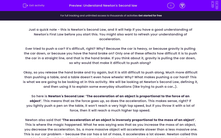# Understand Newton's Second law

In this worksheet, students will use the equation F = ma to understand and apply Newton's Second Law.Key stage:  KS 4

Year:  GCSE

GCSE Boards:   AQA, AQA Trilogy, AQA Synergy, OCR 21st Century, OCR Gateway, Pearson Edexcel, Eduqas,

Curriculum topic:   Forces, Movement and Interactions, Explaining Motion, Motion and Forces, Forces and Motion

Popular topics:   Physics worksheets

Difficulty level:#### Worksheet Overview

Just a quick note - this is Newton's Second Law, and it will help if you have a good understanding of Newton's First Law before you start this. You might also want to refresh your understanding of acceleration.

Ever tried to push a car? It’s difficult, right? Why? Because the car is heavy, or because gravity is pulling the car down, or because you have the hand brake on? Only one of these affects how difficult it is to push the car in a straight line, and that is the hand brake. If you think about it, gravity is pulling the car down, so why would that make it difficult to push along?

Okay, so you release the hand brake and try again, but it is still difficult to push along. Much more difficult than pushing a table, and a table doesn’t even have wheels! Why? What makes pushing a car hard? This is what we are going to be looking at in this activity. We will be looking at Newton's Second Law, defining it, and then using it to explain some everyday situations (like trying to push a car…).

So here is Newton's Second Law: ‘The acceleration of an object is proportional to the force of an object’. This means that as the force goes up, so does the acceleration. This makes sense, right? If you lightly push a pen on the table, it won’t reach a very high top speed, but if you throw it with a lot of force, then it will reach a much higher top speed.

Newton also said that ‘The acceleration of an object is inversely proportional to the mass of an object’. This is where the magic happened. What he was saying was that as you increase the mass of an object,  you decrease the acceleration. So, a more massive object will accelerate slower than a less massive one. This is our car problem – because the car has a lot of mass, it accelerates a lot slower. Newton called this idea inertia -  it is the idea that the heavier the object, the more force is needed to accelerate it.

He went one step further though - he also made an equation with force, acceleration and mass in it:

F = ma

F = force (newtons (N))
m = mass (kilograms (kg))
a = acceleration (metres per second per second (m/s2))

So, let's look at an example together.

Question:  A person is attempting to push a car to get it to accelerate at 2 m/s2. The car has a mass of 900 kg. Calculate the force that will be needed by the person to achieve this acceleration.

Step 1    Highlight the numbers in the question:

A person is attempting to push a car to get it to accelerate at 2 m/s2The car has a mass of 900 kg. Calculate the force that will be needed by the person to achieve this acceleration.

Step 2    Write out the numbers:

F = ?
m = 900 kg
a = 2 m/s2

Step 3   Put them into the equation:

F = 900 x 2

F = 1800 N

Are you ready to have a go at some questions now?

### What is EdPlace?

We're your National Curriculum aligned online education content provider helping each child succeed in English, maths and science from year 1 to GCSE. With an EdPlace account you’ll be able to track and measure progress, helping each child achieve their best. We build confidence and attainment by personalising each child’s learning at a level that suits them.

Get started